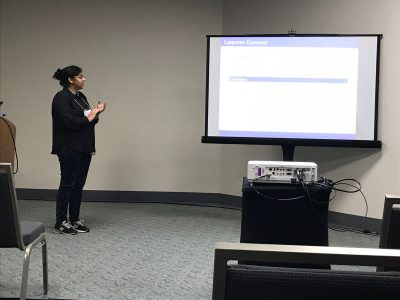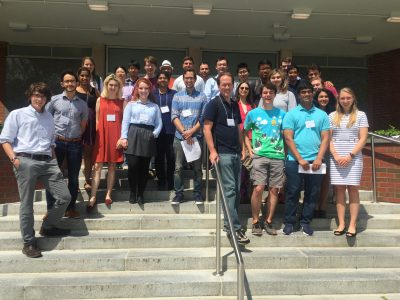# arXiv:1808.03186

Ayelet Amiran, Fabrice Baudoin, Skylyn Brock, Berend Coster, Ryan Craver, Ugonna Ezeaka, Phanuel Mariano, Mary Wishart

project page:

# Probability, Analysis and Mathematical Physics on Fractals 2018

Each year we are looking for a group of undergraduate students to work on Probability, Analysis and Mathematical Physics on Fractals. The aim of the projects will be exploration of differential equations and various operators on fractal domains. Students in the project are supposed to have the usual background in linear algebra and differential equations. Knowledge of Matlab, Mathematica, other computer algebra systems, or programming, as well as proof writing, mathematical analysis, and probability may be helpful but is not required. Previous undergraduate work includes published papers on the eigenmodes (vibration modes) of the Laplacian (2nd derivative) of functions that live on Sierpinski gasket type fractals, and the electrical resistance of fractal networks, as well as work on Laplacians on projective limit spaces. The exact choice of the topics to study will depend on the students’ background and interests. Besides being interesting, taking part in a research project like this may be very useful in the future (for instance, when applying to graduate schools).

# Math UConn REU at JMM 2018

Two of our REU (2017 Stochastics) participants, Raji Majumdar and Anthony Sisti, will be presenting posters Applications of Multiplicative LLN and CLT for Random Matrices and Black Scholes using the Central Limit Theorem on Friday, January 12 at the MAA Student Poster Session, and both of them will be giving talks on Saturday, January 13 at the AMS Contributed Paper Session on Research in Applied Mathematics by Undergraduate and Post-Baccalaureate Students.

Their travel to the 2018 JMM has been made possible with the support of the MAA and UConn’s OUR travel grants.# Financial Math: Portfolio Optimization and Dynamic Programming

## Group Members

Ayelet Amiran, Skylyn Brock, Ryan Craver, Ugonna Ezeaka, Mary Wishart## Overview

Financial markets have asymmetry of information when it comes to the prices of assets. Some investors have more information about the future prices of assets at some terminal time. However, what is the value of this extra information?

We studied this anticipation in various models of markets in discrete time and found (with proof) the value of this information in general complete and incomplete markets. For special utility functions, which represent a person’s satisfaction, we calculated this information for both binomial (complete) and trinomial (incomplete) models.

# arXiv:1808.03186

tba

## Poster

PosterFinMath2018

# 5th Northeast Mathematics Undergraduate Research Mini-Symposium

### University of Connecticut, August 3rd, 2017

#### Participating Schools: Amherst, Smith, UConn and UMass

5th Mini-Symposium full program (2017)## Overview

Laplacians have been well studied on post-critically finite (PCF) fractals. However, less is known about gradients on such fractals. Building on work by Teplyaev, we generalize results regarding the existence and continuity of the gradient on the standard Sierpinski Gasket to higher dimensional Sierpinski Gaskets. In particular, we find that, for functions with a continuous Laplacian, the gradient must be defined almost everywhere, and specify a set of points for which it is defined. Furthermore, we provide a counterexample on higher-dimensional Sierpinski gaskets where the Laplacian is continuous but the gradient is not defined everywhere. We conjecture that Hölder continuity of the Laplacian is a condition strong enough to guarantee that the gradient exists at each point.

# Spectral Analysis on Graphs Related to the Basilica Julia Set

## Overview

We analyze the spectra of a sequence of graphs constructed from the Schreier graphs of the Basilica group.  Our analysis differs from earlier work of Grigorchuk and Zuk in that it is based on a macroscopic decomposition of the graphs. This method gives precise information about the multiplicities of eigenvalues and, consequently, good information about the spectral measures of large graphs. It also permits a proof of the existence of gaps in the spectrum of limiting graphs.

# Spectrum of the Magnetic Laplacian on the Diamond Fractal

## Overview

The diamond fractal is a fractal that is obtained in the following manner.  Start with a graph with two vertices and an edge and replace the edge with two new vertices connected to our original vertices to obtain a diamond shaped graph.   The diamond fractal is defined to be the limiting object after continuing with the edge replacement indefinitely.  In the project, the spectrum of magnetic Laplacian operators on graph approximations to the diamond fractal was computed.

Given a level n approximation to the fractal with known magnetic field strengths through cells and holes, it is possible to determine the net magnetic field through the cells and holes of the preceding graph approximations.  The spectral similarity relation between the operators on successive graph approximations was worked out, with the corresponding spectral decimation polynomial depending on the magnetic field strengths.  A poster and talk on this work was presented at the REU Mini-Symposium at UConn.

# arXiv:1704.01609

## Presentation

Magnetic Spectral Decimation

Poster